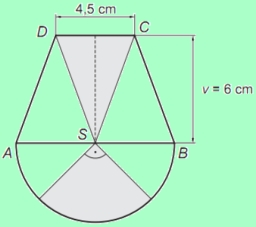# Coat of arms

The class created its coat of arms, which had a shape composed of an isosceles trapezoid ABCD (shorter base is a = 4.5 cm long, longer 2a = 9 cm, trapezoid height 6 cm) and a semicircle with center S and diameter AB. Three identical isosceles triangles formed the trapezoid. Pupils painted half of the semicircle and the middle field of the trapezoid (central triangle) gray. How many were cm2 areas of the coat of arms gray? Round the result to one decimal place.

S =  29.4043 cm2

### Step-by-step explanation:Did you find an error or inaccuracy? Feel free to write us. Thank you!

Tips for related online calculators
Need help calculating sum, simplifying, or multiplying fractions? Try our fraction calculator.
Calculation of an isosceles triangle.